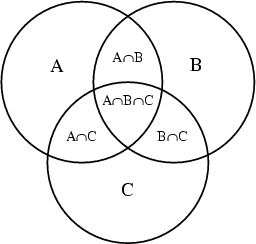# Set Theory - Cardinality (Finite/Infinite Size)

cardinality in set

The cardinality denotes the number of elements or cardinality of the set. (ie its size or length)

## Notation

$$\Large |S|$$

## Example

The sethas a cardinality of 4.

## Type

### Finite

A finite set is a set that you can count.

## Rule

If A and B are finite sets then $$\Large |A \times B| = |A| \times |B|$$ where x denotes the cross product

Discover MoreSet Theory

A set has several interpretation: the original set that does not allow duplicate the multiset (also known as a bag) that allows duplicate This section is what's called the set theory (ie what rules...